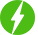# Probability Part – 3

1

Probability Part – 3, Probability.

## Course Description

1. If a card is drawn from a well-shuffled deck of 52 cards, what is the probability of getting different types of cards.

2. If five cards – the ten, jack, queen, king and ace of diamonds, are well-shuffled with their face downwards and one card is picked up at random, what is the probability that the card is queen? If the queen is drawn and put aside, what is the probability that the second card picked up is (a) an ace (b) a queen?

3. If defective pens are accidentally mixed with good ones, then how to calculate the probability that the pen taken out is good one?

4. A lot of bulbs contains some defective bulbs. One bulb is drawn at random. What is the probability that this bulb is defective? If bulb drawn is not defective and not replaced, now if one bulb is picked up, what is the probability that this bulb is not defective?

5. A box contains some discs which are numbered. If one disc is drawn, what is the probability that it bears a two digit number, a perfect square number, a number divisible by 5.

6. A dice has six alphabetic faces, one appearing twice. What is the probability of getting (A) and (D)?

7. If a die is dropped at random on the rectangular region, what is the probability that it will land inside the circle with diameter 1m given in figure?

8. A lot consists of ball pens some of which are defective. Nuri will buy the pen if it is good. What is the probability that she will buy the pen drawn at random by the shopkeeper?

9. A game consists of tossing a one rupee coin 3 times and noting its outcome each time. If the result is three heads or three tails, it means winning. Calculate the probability of losing the game?

10. A die has different numbers some appearing twice. The score obtained on throwing it twice is noted in table. What is the probability that the total score is even, 6, and at least 6?

Free \$39.99
We will be happy to hear your thoughtsRegister New Account
• Total (0)
0